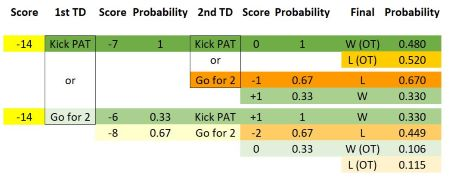# In American football, touchdowns are worth 6 points. After scoring a touchdown, the scoring team...

## Question:

In American football, touchdowns are worth 6 points. After scoring a touchdown, the scoring team may subsequently attempt to score 1 or 2 additional points. Going for 1 point is virtually an assured success, while going for 2 points is successful only with probability p. Consider the following game situation. The Temple Wildcats are losing by 14 points to the Killeen Tigers near the end of regulation time. The only way for Temple to win (or tie) this game is to score two touchdowns while not allowing Killeen to score again. The Temple coach must decide whether to attempt a 1-point or 2-point conversion after each touchdown. If the score is tied at the end of regulation time, the game goes into overtime. The Temple coach believes that there is a 48% chance that Temple will win if the game goes into overtime. The probability of successfully converting a 1-point conversion is 1.0. The probability of successfully converting a 2-point conversion is p.

a. Assume Temple will score two touchdowns and Killeen will not score. Create a decision tree for the decision of whether Temple's coach should go for a 1-point conversion or a 2-point conversion after each touchdown. The terminal nodes in the decision tree should be either WIN or LOSE for Temple.

b. Assume that a WIN results in a value of 1.0 and LOSE results in a value of 0. Further, assume that the probability of converting a 2-point conversion is p = 33%. Should Temple's coach go for a 1-point conversion or 2-point conversion after scoring the first touchdown?

The Temple coach "should go" or "should not go" for 2 points after the first touchdown.

## Decision and Probability Trees:

Decision trees can be constructed using event probabilities and payoffs to identify and select the best strategies when multiple scenarios are possible. A probability tree is a special type of decision tree that maps the possible choices with their associated outcomes and the probability of each outcome. As one decision leads to another decision and a potential outcome, probabilities multiply. The probability tree is complete when all of the potential final outcomes have been identified, with their cumulative probabilities calculated.

a. In setting up our probability tree, the first step is to identify the probability-weighted event outcomes for each touchdown:

• Kick the point after the touchdown (PAT), p = 1.0.
• Fail the 2-point attempt, p = .67.
• Convert the 2-point attempt, p = .33.

Temple's head coach will be faced with two sets of decisions, or nodes. The first decision will be made after the team scores the first touchdown, thereby reducing the deficit from 14 points to 8 points. At that point, Temple can opt for the certainty of 1 point by kicking the PAT, or gamble by going for 2 points.

If Temple kicks the PAT after the first touchdown, the second touchdown will reduce the team's lead to 1 and present Temple with a second decision: send the game to overtime by kicking the PAT, or play for the win in regulation by going for 2.

If Temple goes for 2 after the first touchdown, a successful conversion will reduce the deficit to 6 points, meaning that it will be tied after its second touchdown, at which point the obvious choice is to kick the PAT and win the game in regulation.

If Temple fails the 2-point attempt after the first touchdown, it must convert the 2-point attempt after its second touchdown in order to reach overtime.

The image below depicts each scenario originating from the first decision. The objective is to select the strategy that has the highest probability of winning the game. In the diagram, the winning scenarios are depicted in shades of green. Losing scenarios are highlighted in shades of yellow.

The best strategy for Temple is to play for overtime by kicking each PAT during regulation. Temple can choose the strategy (kicking both PATs) that has a 100% probability of reaching overtime, and then accept its 48% chance of winning the game. Because each PAT is a certainty (p = 1), the odds of winning are dictated only by the odds in overtime.

b. Going for 2 after the first touchdown reduces Temple's chances of a victory to about 43.6%. The team has a 33% chance of winning in regulation by converting the 2-point attempt (p = .33) and then taking the PAT. It also has a 10.6% chance of winning in overtime if it fails the first 2-point attempt (p = .67), convert the second 2-point attempt (p = .33) and then win in overtime (p = .48). The 10.6% probability of this scenario is calculated as .67 x .33 x .48.